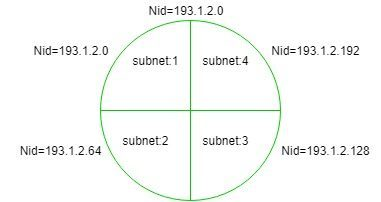# Finding Network ID of a Subnet (using Subnet Mask)

In order to find network id (NID) of a Subnet, one must be fully acquainted with the Subnet mask.

It is used to find which IP address belongs to which Subnet. It is a 32 bit number, containing 0’s and 1’s. Here network id part and Subnet ID part is represented by all 1’s and host ID part is represented by all 0’s.

Example:
If Network id of a entire network = 193.1.2.0 (it is class C IP). For more about class C IP see Classful Addressing.In the above diagram entire network is divided into four parts, which means there are four subnets each having two bits for Subnet ID part.

```Subnet-1: 193.1.2.0 to 193.1.2.63
Subnet-2: 193.1.2.64 to 193.1.2.127
Subnet-3: 193.1.2.128 to 193.1.2.191
Subnet-4: 193.1.2.192 to 193.1.2.255 ```

The above IP is class C, so it has 24 bits in network id part and 8 bits in host id part but you choose two bits for subnet id from host id part, so now there are two bits in subnet id part and six bits in host id part, i.e.,

```24 bits in network id + 2 bits in subnet id = 26 (1's) and
6 bits in host id = 6 (0's) ```

Therefore,

```Subnet Mask = 11111111.11111111.11111111.11000000
= 255.255.255.192```

If any given IP address performs bit wise AND operation with the subnet mask, then you get the network id of the subnet to which the given IP belongs.
Example-1:

```If IP address = 193.1.2.129 (convert it into binary form)
= 11000001.00000001.00000010.10000001
Bit Wise AND  = 11000001.00000001.00000010.10000000
Therefore, Nid = 193.1.2.128```

Hence, this IP address belongs to subnet:3 which has Nid = 193.1.2.128
Example-2:

```If IP address = 193.1.2.67 (convert it into binary form)
= 11000001.00000001.00000010.01000011
Bit Wise AND  = 11000001.00000001.00000010.01000000
Therefore, Nid = 193.1.2.64```

Hence, this IP address belongs to subnet:2 which has Nid = 193.1.2.64My Personal Notes arrow_drop_upCheck out this Author's contributed articles.

If you like GeeksforGeeks and would like to contribute, you can also write an article using contribute.geeksforgeeks.org or mail your article to contribute@geeksforgeeks.org. See your article appearing on the GeeksforGeeks main page and help other Geeks.

Please Improve this article if you find anything incorrect by clicking on the "Improve Article" button below.

Article Tags :
Practice Tags :

3

Please write to us at contribute@geeksforgeeks.org to report any issue with the above content.